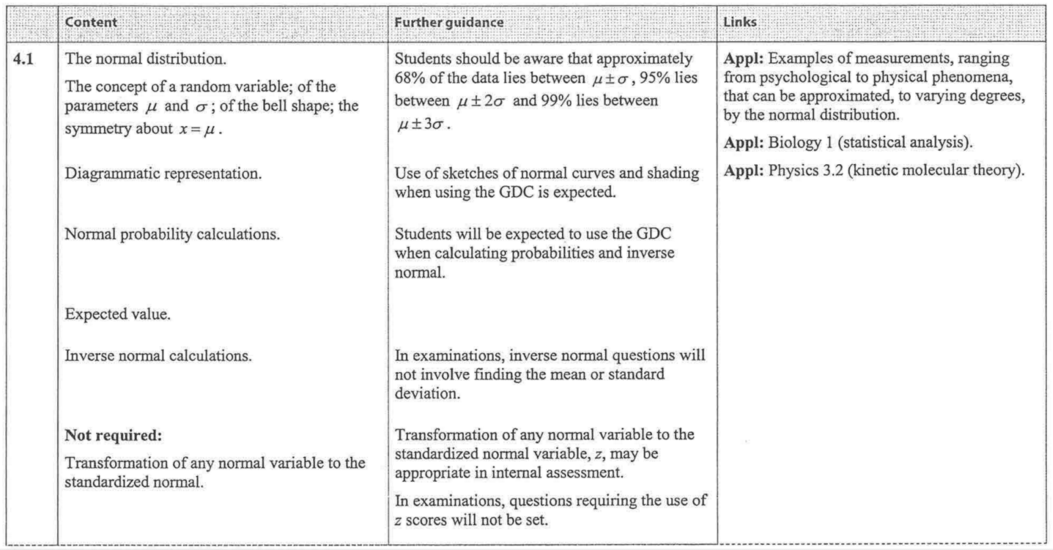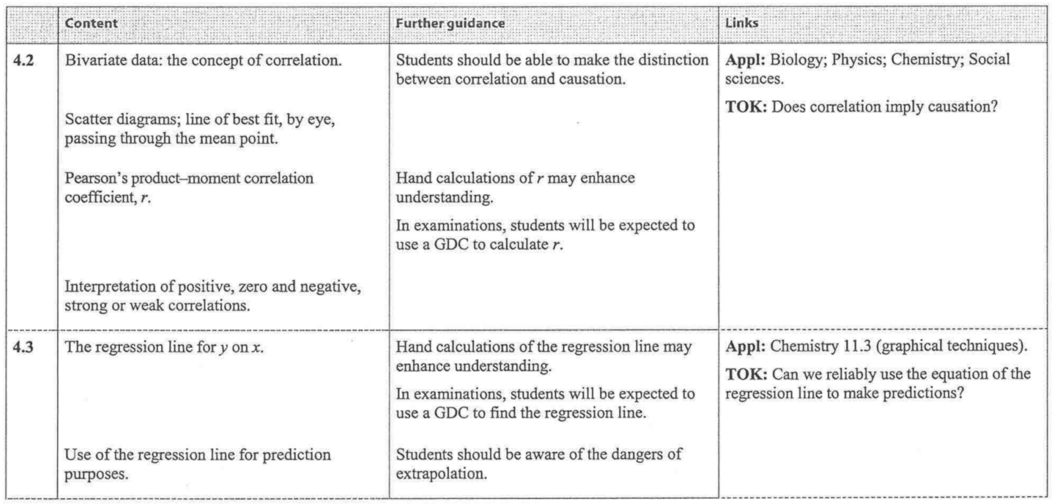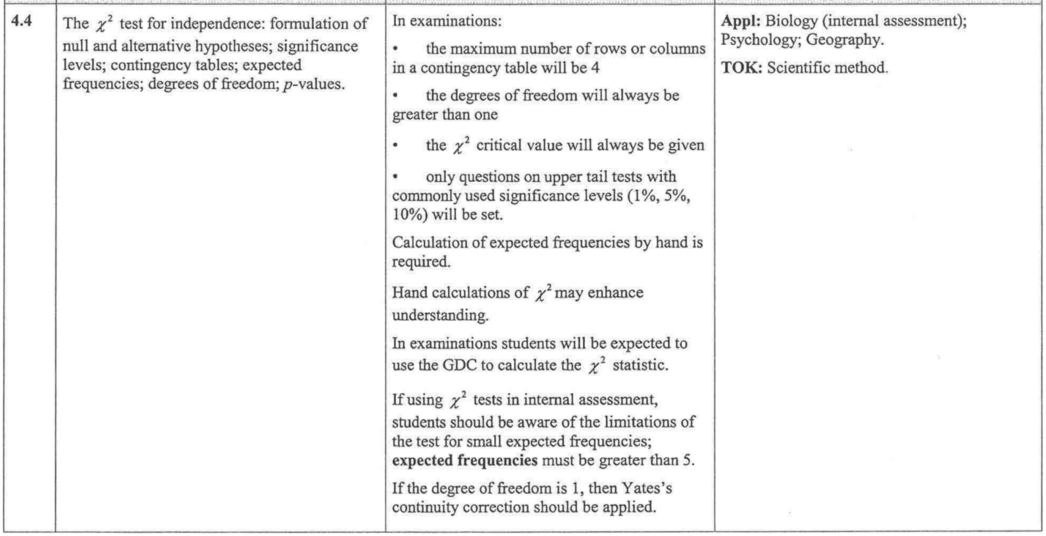### 07. Further Statistics

 IBO Syllabus Topic 4 - Statistical Applications4.1 The normal distributionThe concept of a random variable; of theparameters; of the bell shape; the symmetry.Diagrammatic representation.Normal probability calculations.Expected value.Inverse normal calculations.4.2 Bivarate Data: the concept of correlation4.3 The regression line for y on x4.4 The chi-squared test for independenceTextbook ReferencePrior Learning p 541-5434.1 Statistical GraphsChapter 5 p202-2535.1  The normal distribution p204-216-The properties of a normal distribution- Inverse normal calculations5.2 Correlation p216-228- Types of Correlation- Line of best fit-Pearson's product-moment correlation coefficient5.3 The regression line p228-2325.4 The chi-squared test p233-241Review exercises p242-249Chapter 5 Summary p249-251IBO Formula Booklet-not applicable for this topic-